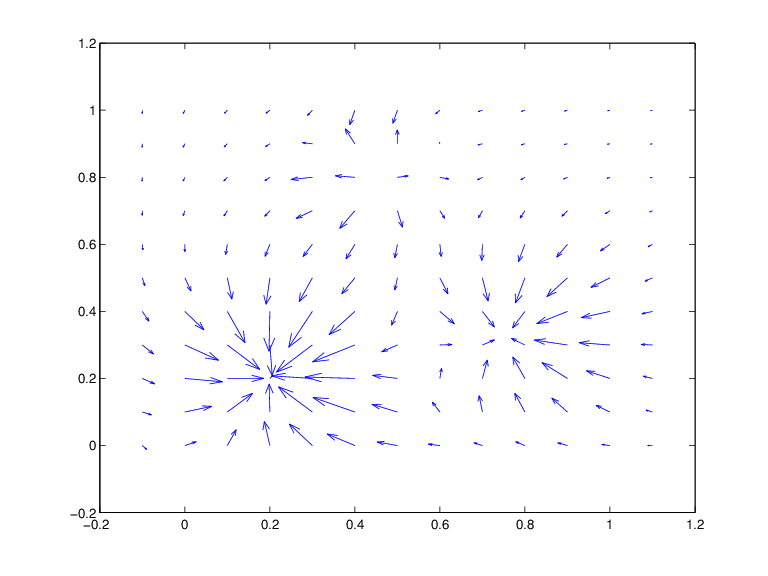Main Content

# fndir

Directional derivative of function

## Syntax

```df = fndir(f,y) ```

## Description

`df = fndir(f,y) ` is the ppform of the directional derivative, of the function f in `f`, in the direction of the (column-)vector `y`. This means that `df` describes the function ${D}_{y}f\left(x\right):={\mathrm{lim}}_{t\to 0}\left(f\left(x+ty\right)-f\left(x\right)\right)/t$.

If `y` is a matrix, with `n` columns, and f is `d`-valued, then the function in `df` is `prod(d)*n`-valued. Its value at x, reshaped to be of size `[d,n]`, has in its jth “column” the directional derivative of f at x in the direction of the jth column of `y`. If you prefer `df` to reflect explicitly the actual size of f, use instead

```df = fnchg( fndir(f,y), 'dim',[fnbrk(f,'dim'),size(y,2)] ); ```

Since `fndir` relies on the ppform of the function in `f`, it does not work for rational functions nor for functions in stform.

## Examples

For example, if `f` describes an `m`-variate `d`-vector-valued function and `x` is some point in its domain, then, e.g., with this particular ppform `f` that describes a scalar-valued bilinear polynomial,

```f = ppmak({0:1,0:1},[1 0;0 1]); x = [0;0]; [d,m] = fnbrk(f,'dim','var'); jacobian = reshape(fnval(fndir(f,eye(m)),x),d,m) ```

is the Jacobian of that function at that point (which, for this particular scalar-valued function, is its gradient, and it is zero at the origin).

As a related example, the next statements plot the gradients of (a good approximation to) the Franke function at a regular mesh:

```xx = linspace(-.1,1.1,13); yy = linspace(0,1,11); [x,y] = ndgrid(xx,yy); z = franke(x,y); pp2dir = fndir(csapi({xx,yy},z),eye(2)); grads = reshape(fnval(pp2dir,[x(:) y(:)].'),... [2,length(xx),length(yy)]); quiver(x,y,squeeze(grads(1,:,:)),squeeze(grads(2,:,:))) ```

Here is the resulting plot.## Algorithms

The function in `f` is converted to ppform, and the directional derivative of its polynomial pieces is computed formally and in one vector operation, and put together again to form the ppform of the directional derivative of the function in `f`.

## Support

#### Machine Learning Challenges: Choosing the Best Classification Model and Avoiding Overfitting

Download white paper